# A Beginner's Guide to Linear Regression Models in R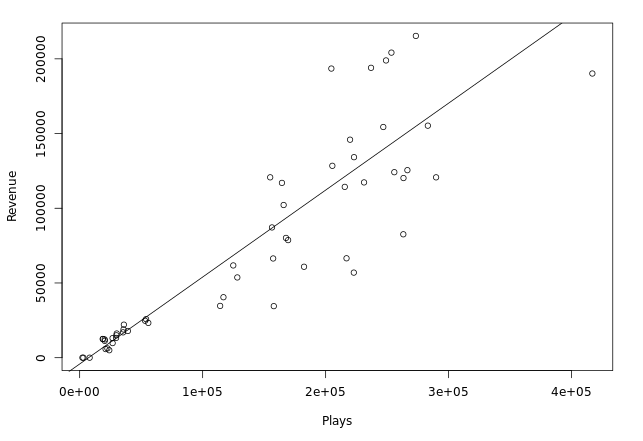New to R? Not a problem! You can still deliver valuable insights to your team using a few simple functions. This article walks through how you can use Periscope's Python and R integration along with its charting capabilities to rapidly build data models.

Here is some information from a fictional gaming company.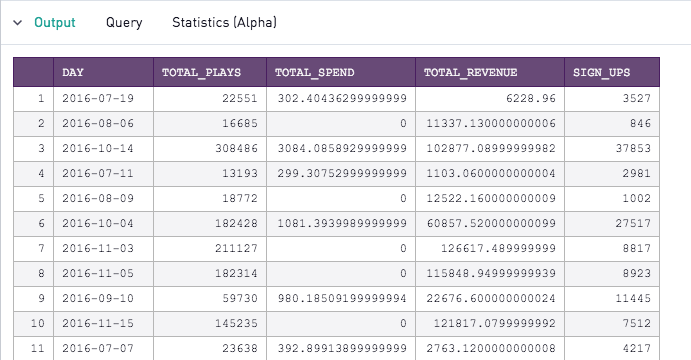That's a lot for a human to parse through. Let's select the "Scatter" chart type in Periscope and click through the different variables to explore possible correlations we can further investigate. Specifically, I want to see if I can predict the revenue for this gaming company based on how many plays have been completed.

Plotting total_plays on the x axis and total_revenue on the y axis, it looks like there's definitely a correlation here worth exploring! Let's dig deeper...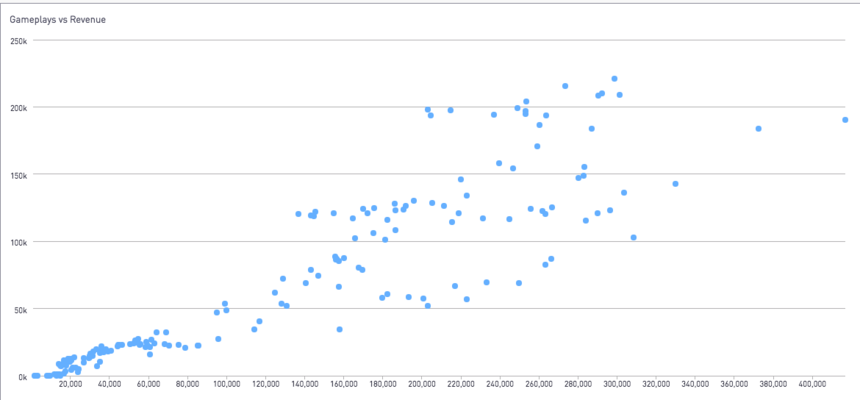There are 2 main steps when creating a model:

1. Building the model on a training data set
2. Testing the model on a testing dataset

This requires us to create 2 subsets of our data. The first subset will be what we use to train our model. Then, we will apply that model onto the second subset. We then can take a look at the difference between the actual values for this subset versus the predicted values.

General guidance suggests that we use 70% of our data in our training dataset, and 30% for testing. It's also imperative that this assignment is random. We will add a column in our SQL output that serves as a flag for which records will be in the training dataset and the test dataset. To create this column, add the following case when statement to your SQL query

```  , case
when random() < 0.3
then 1
else 0
end as test_data_flag```

Great! Now we are ready to get started with R.

In your R editor, first import the dplyr library. This will be useful for splitting the dataframe into a training and test dataset using the filter() call on the test_data_flag field we just created.

```library(dplyr)

# Assigning traning and test data sets based on flag
training <- filter(df, test_data_flag == 0)
test <- filter(df, test_data_flag == 1)
```

Fantastic, now you have your 2 data frames ready for building and testing your model, training and test. Now, let's build the model. R makes this simple, requiring only 1 line of code.

`model <- lm(total_revenue ~ total_plays, data=training)`

Breaking down the above function, we are creating a linear model (lm) with total_revenue as a function of total_plays. The data parameter specifies the name of the dataframe we're using to build the model.

A linear model will be in the form

y*=mx+b

where y* is the predicted value of the response variable (total_revenue) and x is the explanatory variable (total_plays). m is the amount of change in the predicted response with every unit change in the explanatory variable. If you plot x and y*, m is commonly referred to as the slope of the line. b is the predicted y* when x=0. On a graph, this would be the y intercept.

To see what the m and b are for this linear model, call summary(model). You can select your chart type as "R Text" to see this output and print it on your dashboard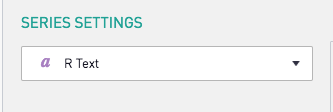Here's our output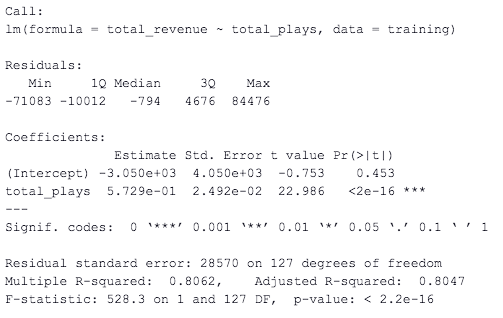Let's take a closer look at the "Coefficients" section first under the "Estimate" column. The estimated y intercept is -3050, and the estimated change in the predicted revenue as a function of total_plays is 0.5729. Note that the y intercept doesn't make much intuitive sense here (this implies we have negative revenue when there are 0 gameplays). It's important to take any model outputs with a grain of salt, and to understand when it makes sense to use the model, versus when another approach would be more practical.

We also want to inspect the Pr(>|t|) in the "total_plays" row; this indicates how strong of a correlation there is between the two variables. We want a result as close to 0 as possible, which definitely seems to be the case here (less than 2 * 10^-16)

The residuals section at the top shows the spread between the actual total_revenue and the predicted total_revenue. We will take a closer look at residuals in our test data in the next step.

Now that we've created a model. It's time to test it! Again, this is a quick line of code in R

`test\$pred <- predict(model, test)`

Here, we are creating a column on our test data frame that contains the predicted values using the model we just created. While we are at it, let's also calculate the residuals (the difference between the actual total_revenue and the predicted values from our model)

`test\$residuals = test\$total_revenue - test\$pred`

Now we output the result

`periscope.table(test)`

Take a look at your output. We have successfully created columns with our model's predicted values for the test values, and also computed the residuals for each record!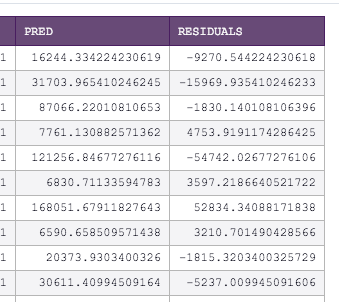Now let's say you want to plot your linear regression line against your test data. Take out the periscope.table(test) line and write the following lines of code, and select your chart type as R Image

```linear_regression_plot <- plot(test\$total_plays,test\$total_revenue, xlab = "Plays", ylab="Revenue")
abline(model)

periscope.image(linear_regression_plot) ```

This gives the below result: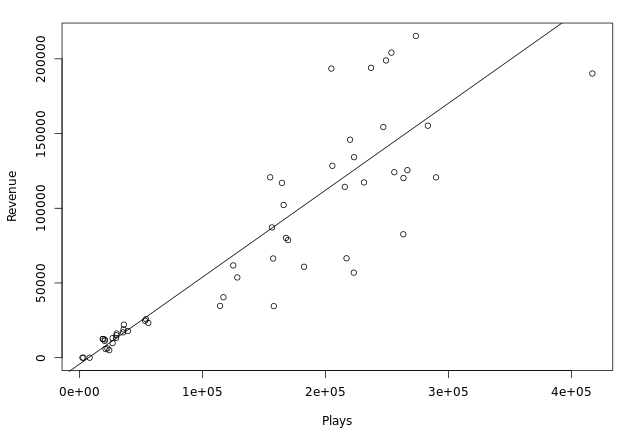Now, we want to plot our residuals. The goal of this exercise is to ensure that there isn't any sort of trend between the x value and the residuals. This would indicate that a non-linear regression model would be better suited to explain the relationship between these 2 variables.

First, revert the periscope.image(linear_regression_plot) line back to:

`periscope.table(test)`

Then, set the following visualization options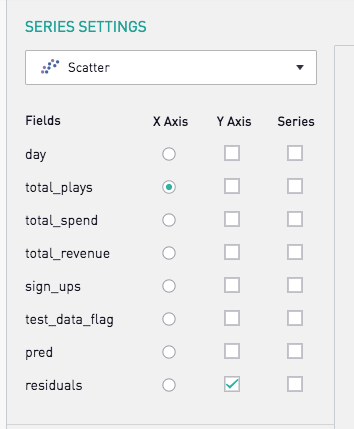And there we have it. A residual plot that shows an effectively random distribution between the residuals and the x values. This makes a strong case that there exists a linear relationship between total_plays and total_revenue.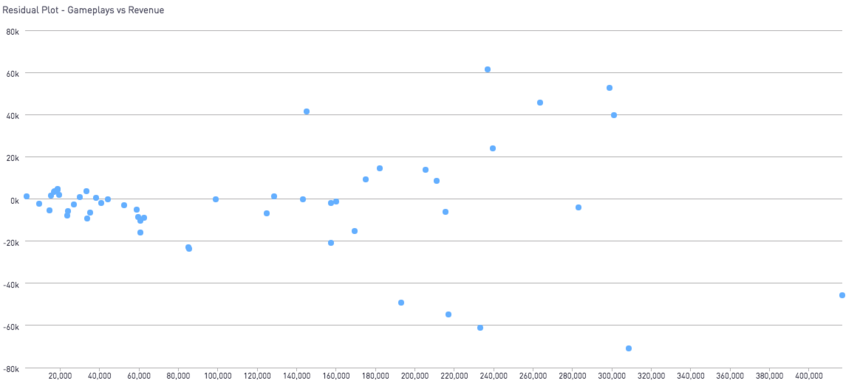Once you've gotten a grasp on bivariate regressions (one x and one y), try your hand at multivariate regressions!

New to R? Did you find this post helpful? Let us know in the comments what you'd like to see!

For more information on linear regression models in R, I found this documentation from R to be especially helpful!

Prefer Python? Check out the community post here for an equivalent example.